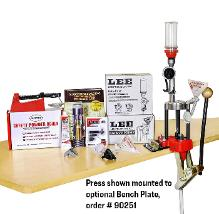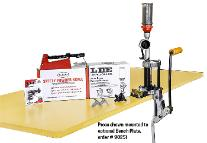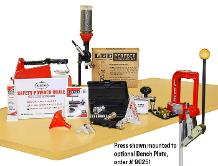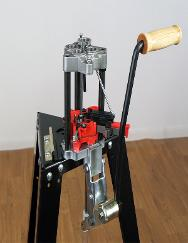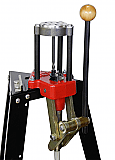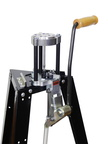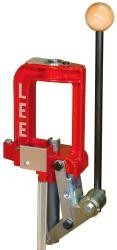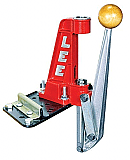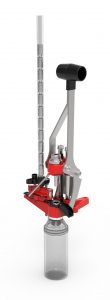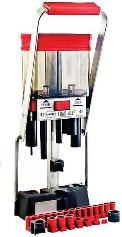MOLDS

90286 – TL452-230-TC = \$48

90317 – 358-140-SWC = \$48

90387 – 356-120-TC = \$48

90690 – 401-175-TC = \$48

90028 – #4 buckshot mold = \$55

90289 – 452 – 230 – TC  = \$48

90306 – 358 – 125 – RF = \$48

90326 – 358 – 150 -1R = \$48

90387 – 356 – 120 – TC = \$48

90459 – C225 – 55 – RF = \$48

90692 – 358 – 158 – RF = \$48

90697 – 452 – 200 – RF = \$48

90268 – 459 – 405 HB = \$30

90338 – C429 – 240 – SWC = \$25

90351 – 452 – 228 – 1R = \$25

90305-356-102-1R = \$25

90402 – TL356-124-TC = \$48

90433 – TL401-175SWC = \$48

90451 – C225-55-RF = \$25

90457 – 356-125-2R = \$48

90464 – TL356-124-2R = \$25

90486 OO Buckshot = \$55

90238 TL 356-124-TC = \$25

90239 356-120-TC = \$25

90281 1 OZ. Slug = \$35

90463 TL452-200-SWC = \$25

90362 C309-113-F = \$25

90366 C309-150-F = \$25

90404 58 cal. – 440 = \$25

90975 Ball 600 = \$25

90359 C452-300-RF = \$25

90398 54 cal. – 300 = \$25

90672 TL356 – 95 – RF = \$25

90346 TL 452-230-2R = \$25
90192 – OOO Buckshot mold = \$55
Commercial mold handles = \$20

Meltors

Lee Production Pot IV = \$70
Lee Pro 4-20 = \$85

Lee Misc.

Chamfer Tool = \$4

Lee Manual = \$20

Lee Ultimate Lock Rings = \$9                                            Lee Lock Rings = \$4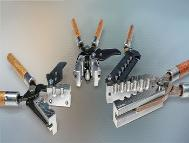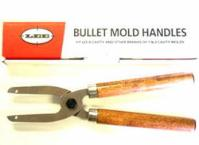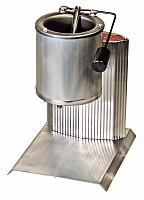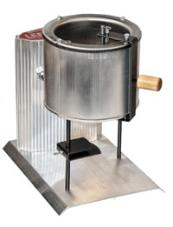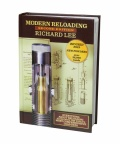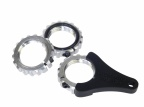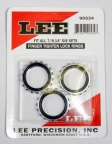Press Kits & Presses

Lee Auto Breech Lock Pro Press Only = \$140

Lee 4 hole Turret Press = \$106

Lee 4 hole Turret Press Kit = \$182

Lee Classic Turret Press = \$151

Lee Classic Turret Press Kit = \$265

Lee Breechlock Challenger Press = \$80
Lee Breechlock Challenger Kit = \$160

Lee APP Press = \$91
Lee APP Swage Kit = \$38                                                    Lee 20 gauge Load-All II = \$60

Powder Measures

Deluxe Perfect Powder Measure = \$65

Lee Auto Drum Powder Measure = \$42

Pro Auto Disk Powder Measure = \$44

Lee Perfect Powder Measure = \$27

Primer Tools

Lee Auto Bench Prime = \$35
Lee Auto Prime XR = \$25
Lee Ergo Prime = \$40
Set Auto Prime Shellholder kit = \$20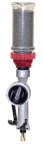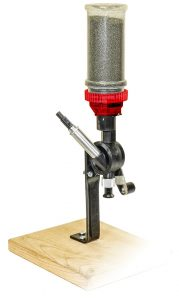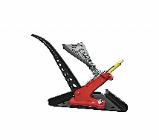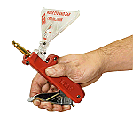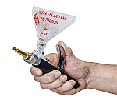Dies

Pistol

45 ACP Deluxe die set = \$55

25 ACP die set = \$35

38 Special/357 Magnum Deluxe die set = \$55

38/357 3 Die Set = \$35

9mm Deluxe die set = \$55

9mm 3 die set = \$35

380 Deluxe die set = \$55

380 3 die set = \$35

40 S&W 3 Die set = \$35

44 Deluxe Die Set = \$55

44 3 die set = \$35

45 Colt Deluxe die set = \$55

45 Colt 3 Die Set = \$35

500 S&W Magnum Die set= \$35

Pistol Carbide Factory Crimp

9mm Carbide Factory Crimp die = \$22

380 Auto Carbide Factory Crimp die = \$22

Rifle

300 Blackout die set = \$35

30/06 Pacesetter die set = \$35

308 Ultimate die set = \$55

6.5 Creedmoor RGB die set = \$22

375 Winchester die set = \$35

30/40 Krag die set = \$35

38-55 die set = \$35

6.5 Carcano die set = \$35

7.35 Carcano die set = \$35

270 Winchester Pacesetter Die set = \$35

224 Valkyrie Die Set = \$35

223 pacesetter die set = \$35

45-70 die set = \$35

5.7×28 2 die set = \$35

243 Ultimate die set =\$55

350 Legend die set = \$35

6.5 Creedmoor Ultimate Die set = \$55

300 Savage pacesetter die set = \$35

22/250 pacesetter die set = \$35

25-20 pacesetter die set = \$35

Rifle Factory Crimp

375 Winchester Factory crimp die = \$13

35 Remington Factory Crimp die = \$13

45/70 Factory Crimp die = \$13

5.7×28 FN Factory Crimp die = \$20

308 Factory Crimp die = \$13

30/06 Factory Crimp die = \$13

223 Factory Crimp die = \$13

Collet

223 Collet die only = \$23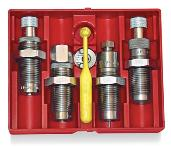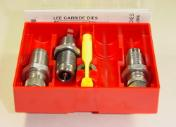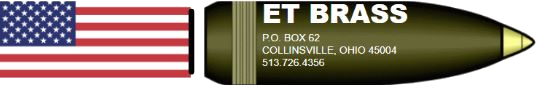Scroll to top